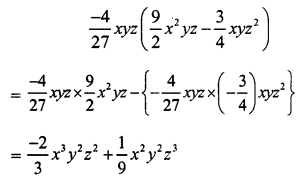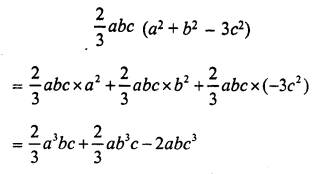# RS Aggarwal Class 7 Solutions Chapter 6 Algebraic Expressions Ex 6C

In this chapter, we provide RS Aggarwal Solutions for Class 7 Chapter 6 Algebraic Expressions Ex 6C for English medium students, Which will very helpful for every student in their exams. Students can download the latest RS Aggarwal Solutions for Class 7 Chapter 6 Algebraic Expressions Ex 6C Maths pdf, free RS Aggarwal Solutions Class 7 Chapter 6 Algebraic Expressions Ex 6C Maths book pdf download. Now you will get step by step solution to each question.

### RS Aggarwal Solutions for Class 7 Chapter 6 Exponents Ex 6C Download PDF

Question 1.
Solution:
4a(3a + 7b) = 4a x 3a + 4a x 7b = 12a2 + 28ab

Question 2.
Solution:
5a(6a – 3b) = 5a x 6a – 5a x 3b = 30a2 – 15ab

Question 3.
Solution:
8a(2a + 5b) = 8a2 x 2a + 8a2 x 5b = 16a3 + 40a2b

Question 4.
Solution:
9x2 (5x + 7) = 9x2 x 5x + 9x2 x 7 = 45x3 + 63x2

Question 5.
Solution:
ab(a2 – b2) = ab x a2 – ab x b2 = a3b – ab3

Question 6.
Solution:
2x2 (3x – 4x2) = 2x2 x 3x – 2x2 x 4x2 = 6x3 – 8x4

Question 7.
Solution:Question 8.
Solution:
-1 7x2 (3x – 4) = -17x2 x 3x – 17x2 x (-4) = -51x3 + 68x2

Question 9.
Solution:Question 10.
Solution:
-4x2y (3x2 – 5y)
= -4xy x 3x2 – 4xy x (-5y)
= -12xy + 20xy2

Question 11.
Solution:Question 12.
Solution:
9t2 (t + 7t3) = 9t2 x t + 9t2 x 7t3 = 9t3 + 63t5

Question 13.
Solution:Question 14.
Solution:Question 15.
Solution:Question 16.
Solution:
24x2 (1 – 2x)
= 24x2 x 1 – 24x2 x 2x
= 24x2 – 48x3
If x = 2, then
24x2 – 48x3
= 24(2)2 – 48(2)3
= 24 x 4 – 48 x 8
= 96 – 384
= -288

Question 17.
Solution:Question 18.
Solution:
s (s2 – st) = s x s2 – s x st = s3 – s2t
If s = 2, t = 3, then
s3 – s2t = (2)3 – (2)2 x 3 = 8 – 4 x 3 = 8 – 12 = -4

Question 19.
Solution:
-3y (xy + y2) = -3y x xy + (-3y) x y2 = -3xy2 – 3y3
if x = 4, y = 5, then
-3xy2 – 3y3
= -3(4)(5)2 – 3(5)3 = -3 x 4 x 25 – 3 x 125 = -300 – 375 = -675

Simplify each of the following:
Question 20.
Solution:
a(b – c) + b(c – a) + c(a – b) = ab – ac + bc – ab + ac – bc = 0

Question 21.
Solution:
a(b – c) – b(c – a) – c(a – b) = ab – ac – bc + ab – ac + bc = 2ab – 2ac

Question 22.
Solution:
3x2 + 2(x + 2) – 3x (2x + 1)
= 3x2 + 2x + 4 – 6x2 – 3x = 3x2 – 6x2 + 2x – 3x + 4 = -3x2 – x + 4

Question 23.
Solution:
x (x + 4) + 3x (2x2 – 1) + 4x2 + 4
= x2 + 4x + 6x3 – 3x + 4x2 + 4
= 6x3 + x2 + 4x2 + 4x – 3x + 4
= 6x3 + 5x2 + x + 4

Question 24.
Solution:
2x2 + 3x (1 – 2x3) + x (x + 1)
= 2x2 + 3x – 6x4 + x2 + x
= – 6x4 + 2x2 + x2 + 3x + x
= – 6x4 + 3x2 + 4x

Question 25.
Solution:
a2b (a – b2) + ab(4ab – 2a2) – a3b (1 – 2b)
= a3b – a2b3 – 2a3b2 + 4a2b3 – 2a3b2 – a3b + 2a3b2
= a3b – a3b + 2a3b2 – a2b3 + 4a263
= 3a2b3

Question 26.
Solution:
4st (s – t) – 6s2 (t – t2) – 3t2 (2s2 – s) + 2st(s – t)
= 4s2t – 4st2 – 6s2t + 6s2t2 – 6s2t2 + 3st2 + 2s2t – 2st2
= 4s2t – 6s2t + 2s2t – 4st2 + 3st2 – 2st2 + 6s2t2 – 6s2t2
= 6s2t – 6s2t – 6st2 + 3st2 + 6s2t2 – 6s2t2
= – 3st2

All Chapter RS Aggarwal Solutions For Class 7 Maths

—————————————————————————–

All Subject NCERT Exemplar Problems Solutions For Class 7

All Subject NCERT Solutions For Class 7

*************************************************

I think you got complete solutions for this chapter. If You have any queries regarding this chapter, please comment on the below section our subject teacher will answer you. We tried our best to give complete solutions so you got good marks in your exam.

If these solutions have helped you, you can also share rsaggarwalsolutions.in to your friends.